NEET  >  DC Pandey Solutions: Wave Motion

# DC Pandey Solutions: Wave Motion - Notes | Study Physics Class 11 - NEET

 Table of contentsIntroductory Exercise 14.1Introductory Exercise 14.2Introductory Exercise 14.3Introductory Exercise 14.41 Crore+ students have signed up on EduRev. Have you?

Introductory Exercise 14.1

Q.1. Prove that the equation y = a sin wt does not satisfy the wave equation and hence it does not represent a wave.
Sol.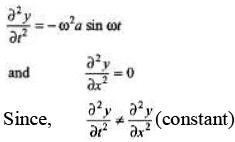Hence, the given equation does not represent a wave equation.

Q.2. A wave pulse is described by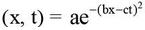, where a, b and c are positive constants. What is the speed of this wave?
Sol.

Speed of wave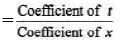= c/b

Q.3. The displacement of a wave disturbance propagating in the positive x-direction is given by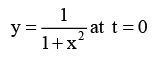and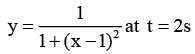where, x and y are in metre. The shape of the wave disturbance does not change during the propagation. What is the velocity of the wave?
Sol.

At t = 0, y is maximum at x = 0.
At t = 2 s, y is maximum at x = 1 m.
Hence, in 2 s, wave has travelled 2m in positive x-direction.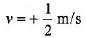= +0.5 m/s

Q.4. A travelling wave pulse is given by,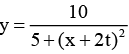Here, x and y are in metre and t in second. In which direction and with what velocity is the pulse propagating? What is the amplitude of the pulse?

Sol. Since the coefficient of t and x are opposite signs, the wave is travelling along negative x-direction. Further,
Speed of wave =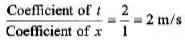Amplitude = maximum value of y
= 10/5 = 2m

Q.5. If at f = 0, a travelling wave pulse on a string is described by the function,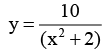Here x and y are in metre and t in second. What will be the wave function representing the pulse at time t, if the pulse is propagating along positive x-axis with a speed of 2 m/s?

Sol.  Since the wave is travelling along positive x-direction. Hence, the coefficient of t and coefficient of x should have opposite signs. Further∴  Coefficient of t = 2 (coefficient of x) = 2 x 1 = 2 SI units.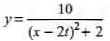Introductory Exercise 14.2

Q.1. The equation of a travelling wave is,
y(x, t ) 0.02 sin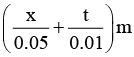Find: (a) The wave velocity and
(b) the particle velocity at x = 0.2 m and t = 0.3 s.
Given cos θ = -0.85 where θ = 34 rad
Sol.

(a) Wave velocity =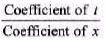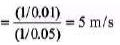Since, coefficient of t and coefficient of x are of same signs. Hence, wave is travelling in negative x-direction.
or
v = -5 m/s
(b) Particle velocity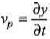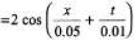Substituting x = 0.2 and t = 0.3, we have Vp = 2cos(34)
= (2) (-0.85) =-1.7 m/s

Ques 2: Is there any relationship between wave speed and the maximum particle speed for a wave travelling on a string? If so, what is it?
Sol:
As we know, Wave speed,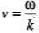and maximum particle speed, (vp)max = wA
From these two expressions, we can see that,
(vp)max = (kA)V

Q.3. Consider a sinusoidal travelling wave shown in the figure. The wave velocity is + 40 cm/s. Find :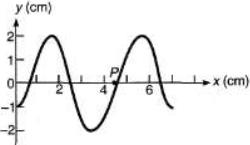(a) the frequency
(b) the phase difference between points 2.5 cm apart
(c) how long it takes for the phase at a given posit ion to change by 60°
(d) the velocity of a particle at point P at the instant shown.
Sol.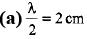⇒ λ = 4cm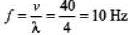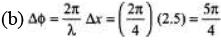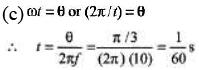(d) At P, particle is at mean position. So, v = maximum velocity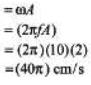= 125.7 cm/s= 1.26 m/s
Further,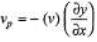...(i)
Sign of v1, the wave velocity is given positive.
Sign of dy/dx, slope of y - x graph is also positive.
Hence, from Eq. (i) particle velocity is negative. vp = -1.26 m/s

Q.4. Transverse waves on a string have wave speed 12.0 m/s, amplitude 0.05 m and wavelength 0.4 m. The waves travel in the + x direction and at t = 0 the x = 0 ends of the string has zero displacement and is moving upwards.
(a) Write a wave function describing the wave.
(b) Find the transverse displacement of a point at x = 0.25 m at time t = 0.15 s.
(c) How much time must elapse from the instant in part (b) until the point at x = 0.25 m has zero displacement?
Sol.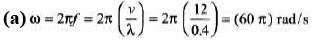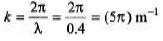Since wave is travelling along + ve x-direction, at and kx should have opposite signs. Further at t = 0, x = 0 the string has zero displacement and moving upward (in positive direction).
Hence at x = 0, we should have Asinwt not - Asinwt. Therefore, the correct expression is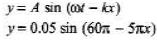(b) Putting x = 0.2 m  and  t = 0.15 s  in the above equation we have,
y= 0.0354 m = 3.54 cm
(c) In part (b), y = A/√2
From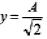to y = 0, time taken is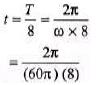= 4.2 × 10-3s = 4.2 ms

Introductory Exercise 14.3

Q.1. Calculate the velocity of a transverse wave along a string of length 2 m and mass 0.06 kg under a tension of 500 N.
Sol.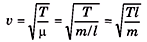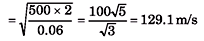Q.2. Calculate the speed of a transverse wave in a wire of 1.0 mm2 cross-section under a tension of 0.98 N. Density of the material of wire is 9.8 × 103 kg/m3.
Sol.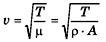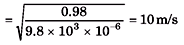Introductory Exercise 14.4

Q.1. Spherical waves are emitted from a 1.0 W source in an isotropic non-absorbing medium. What is the wave intensity 1.0 m from the source?
Sol.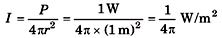Q.2. A line source emits a cylindrical expanding wave. Assuming the medium absorbs no energy, find how the amplitude and intensity of the wave depend on the distance from the source?

Sol. For line source,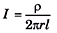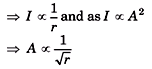The document DC Pandey Solutions: Wave Motion - Notes | Study Physics Class 11 - NEET is a part of the NEET Course Physics Class 11.
All you need of NEET at this link: NEET

## Physics Class 11

127 videos|464 docs|210 tests
 Use Code STAYHOME200 and get INR 200 additional OFF

## Physics Class 11

127 videos|464 docs|210 tests

### How to Prepare for NEET

Read our guide to prepare for NEET which is created by Toppers & the best Teachers

Track your progress, build streaks, highlight & save important lessons and more!

,

,

,

,

,

,

,

,

,

,

,

,

,

,

,

,

,

,

,

,

,

;